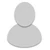## FANDOM

3,876 Pages

• I was wondering how you would calc a planet being sub-atomically destroyed (how it interacts with GBE and the J/cc), for example, the Earth

• We use j/cc.

Basically find the volume of the planet, then subtract the ocean and core and then use the subatomize values for each element.

• Let's see here,

Volume of Earth: 1 trillion cubic kilometers (Google)

Volume of core: 15% (Google), or 150 Billion cubic kilometers

Volume of oceans: 1.332 Billion Km^2 (Google, you're weird)

Convertions: 10^15 due to being cubed. Earth: 10^27 cm^2, core: 1.5e+26, oceans: 1.332e+24

So add the ocean and core (to make subtraction simpler): 1.51332e+26

10^27 - 1.51332e+26 = 8.48668e+26 cm^2

Subatomize value: 5.403e+13 J/cc (Doing this one because I don't feel like trying to find the % of materials the Earth is made of right now), 8.48668e+26*5.403e+13 = 4.5853532e+40 J. Small Star level

Hope I did that right.

• What I meant was you should use the value of subatomization for water for the ocean and iron for the core, then basic value for the rest of the planet. But yeah, Low 4-C is around the genera result.

•